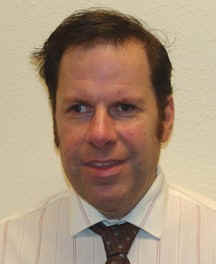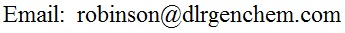﻿ Select Semester to View

# GENERAL CHEMISTRY PAGESWelcome to Mr. Robinson's General Chemistry Pages. The content of this site is maintained by Dale L. Robinson, Assistant Professor of Chemistry. The primary purpose of this site is to assist students in Mr. Robinson's classes, but all visitors are welcome. If you have questions or comments about this site, please email Mr. Robinson at:To guard against spambots, the above email address is not clickable.  But you can write down the address and physically type it into your email client to send me a message.

Current Date and Time in San Antonio, Texas:

Web-based Calculator:

To view semester-specific content, select a semester to view from the links below.  Below the semester links (staying on this page) you will find general content that remains useful semester after semester.  This includes things like practice problems, and general chemistry lecture notes.

Summer 2020

GRAPHS THAT ILLUSTRATE CHEMICAL CONCEPTS

Time evolution of evaporation and condenstation rates for a liquid in a closed containerVapor Pressure of ideal and non-ideal solutionsAverage, Instantaneous and Initial RatesGENERAL CHEMISTRY LECTURE NOTES  (NEW or REVISED)

Colligative Properties (SPRING 2015)

GENERAL CHEMISTRY LECTURE NOTES  (ORIGINAL)

 Scientific Method (PowerPoint Slides) Unit Conversions Significant Figures  (PowerPoint Slides) Classification of Matter Chemical Nomenclature  (PowerPoint Slides) Counting Atoms in Chemical Formulas Writing and Balancing Chemical Equations Stoichiometry Classifying Chemical Reactions Detailed Development of the Gas Laws Summary of Gas Law Calculations Acid / Base Neutralization Reactions Thermochemistry Electronic Structure Part 1 (Bohr Model) Electronic Structure Part 2 (Quantum Energy Levels) States of Matter (PowerPoint:  1  2  3) The Chemistry of Solutions Chemical Kinetics Chemical Equilibrium (General Principles) Solids and Liquids in Chemical Equilibria Acid / Base Equilibria Solubility Equilibria Thermochemistry Powerpoint Slides

MR. ROBINSON'S POWERPOINT PRESENTATIONS

Intro and Scientific Method

Significant Figures

What is Chemistry?
Concepts covered:  Mixtures, substances, elements, and compounds.  Laws of chemical combination, including the law of definite proportions, the law of multiple proportions, and conservation of mass.  Also, a look at the evidence that lead to our current model of the atom, the nuclear model.

Vaporization
Covers evaporation of liquids from open containers, the establishment of equilibrium vapor pressure in closed containers, boiling of liquids, and phase diagrams.

Crystal Structure
Covers the cubic crystal structures, which include simple cubic (also known as primative cubic), body-centered cubic, and face-centered cubic (also know as cubic close-packed)

Solutions
This is the PowerPoint set presented in class while covering Chapter 12, on the chemistry of solutions.

Chemical Kinetics
This is one of the PowerPoint sets presented in class while covering chemical kinetics.

Chemical Kinetics
This is one of the PowerPoint sets presented in class while covering chemical kinetics.

Acid-Base Equilibria

OLD EXAMS -- CHEM 1311

CHEM 1311.001 EXAM 1 from Summer I 1998 (Friday June 12, 1998)

CHEM 1311.001 EXAM 1 Friday September 18, 1999
This is a 40-question exam covering the scientific method, significant figures, the metric system, scientific notation, and conversion factors
Covers:
* Definitions of terms related to the scientific method (prob 1-6)
* Practical application of terms related to the scientific method (prob 7-10)
* Concepts related to the use of conversion factors (prob 11-13)
* Floating point and scientific notation numbers and conversions between these formats (prob 14-17)
* The metric system (prob 18)
* Unit conversion calculations (prob 19-20)
* Significant figures in measurements and calculations; rules and concepts (prob 21-40)

CHEM 1311.001 EXAM 1 Friday February 12, 1999
This is a 40-question exam covering the scientific method, significant figures, classification of matter, and chemical nomenclautre
Covers:
* Scientific method -- basic definitions and practical applications (prob 1-7)
* Significant figures in data and calculation; rules and concepts (prob 8-21)
* Classification of matter (practical application) (prob 22-23)
* Formulas of ionic compounds containing only monatomic ions (prob 24-29)
* Formulas of ionic compounds containing polyatomic ions (prob 30-34)
* Names and formulas of ionic compounds containing transition metal cations (prob 35-38)
* Names of binary and oxoacids (prob 39-40)

CHEM 1311.001 EXAM 2 Friday October 16, 1998
Covers definitions of Element, Compound, Substance, Mixture (Prob. 1-4)
Covers practical application of definitions in 1-4 (Prob. 5-10)
Covers formulas of ionic compounds containing only monatomic ions (Prob. 11-20)
Covers formulas of ionic compounds containing polyatomic ions (Prob. 21-25)
Covers formulas of ionic compounds containing transition metal cations (Prob. 26-30)
Covers Classification of reactions by type (Prob. 31-37)
Covers predicting when precipitates will form (Prob. 38-40)

CHEM 1311.002 EXAM 3 Thursday November 12, 1998
Covers molecular, ionic, and net ionic equations (Prob. 1-17)
Covers mass percentages, empirical and molecular formulas, and stoichiometry (Prob. 18-25)

CHEM 1311.001 EXAM 2 Friday October 17, 1997
Covers formulas of ionic compounds containing only monatomic ions (Prob. 1-5)
Covers formulas of ionic compounds containing polyatomic ions (Prob. 6-8)
Covers mass percentages and empirical formulas (Prob. 9-10)

CHEM 1311.001 EXAM 2 Friday March 5, 1999
This is a 20-question exam about spectator ions, net ionic equations, and classifying reactions.
* Covers basic spectator ion concepts (prob 1-2)
* Covers predicting precipitate formation (prob 3-6)
* Covers spectator ions and net ionic equations (prob 7-10)
* Covers spectator ions in acid-base neutralization reactions (prob 11-13)
* Covers acid-base neutralization concepts (prob 14-15)
* Covers classifying reactions by type (prob 16-17)
* Covers vocabulary related to reaction types (prob 18-19)
* Covers classifying reactions by type (prob 20)

CHEM 1311.001 EXAM 3 Friday November 14, 1997
Covers the gas laws. This includes the empirical gas laws, the ideal gas law and its variants (such as the gas density formula).

CHEM 1311.003 Exam 2 Fall 1993
Covers:
* Empirical formula calculation (using mass percentage data)
* Stoichiometry with limiting reactant concept
* Balancing chemical equations
* Gas law calculations
* Water solubility rules for ionic compounds
* Identifying spectator ions
* Predicting precipitate formation
* Classifying chemical reactions by type
SPECIAL FEATURES: A link is provided at the end of the test to a copy with the answers marked in bold type. On the version with the answers, selected problems have a link to a graphic image (jpg file) of the instructor's handwritten solution

CHEM 1311.001 EXAM 3 Monday March 29, 1999
This is a 14-question exam covering stoichiometry of chemical formulas and chemical reactions.
* Covers calculation of mass percentages from a chemical formula (prob 1)
* Covers calculation of empirical formulas from percentage and mass data (prob 2-3)
* Covers stoichiometry of chemical reactions where the limiting reactant is known (prob 4-6)
* Covers general concepts of limiting reactants (non-mathematical) (prob 7-9)
* Covers stoichiometry of chemical reactions where the limiting reactant is not initially known (prob 10-13)
* Covers the percent yield concept (prob 14)

CHEM 1311.001 EXAM 4 Wednesday April 28, 1999
This is a 20-question exam about the gas laws
* Covers terminology and basic concepts (non-mathematical) (prob 1-9)
* Covers calculations involving the empirical gas laws (prob 10-14)
* Covers calculations involving the Ideal Gas Law and its variants (prob 15-20)

CHEM 1311.001 EXAM 4 Thursday July 2, 1998
This is a 20-question exam about thermochemistry
* Covers terminology (endothermic, exothermic) and basic principles (prob 1-7)
* Covers use of formula q = s . m . Dt (prob 8-12)
* Covers Hess' Law (prob 13-19)
* Covers Calorimetry (prob 20)

CHEM 1311.001 EXAM 4 Thursday July 3, 1997
This is a 16-question exam about thermochemistry
* Covers terminology (endothermic, exothermic) and basic principles (prob 1-4)
* Covers use of formula q = s . m . Dt (prob 5-9)
* Covers Hess' Law (simple) (prob 10-12)
* Covers Thermochemical Stoichiometry (prob 13)
* Covers Calorimetry (prob 14)
* Covers Hess' Law (advanced) (prob 15-16)

CHEM 1311.001 EXAM 4 Fri. Dec. 5, 1997
This is a 10-question exam about thermochemistry
Covers:
* Terminology and basic concepts (prob 1-3)
* Use of the formula q = s . m . Dt (prob 4-6)
* Advanced use of Hess' law to calculate DH for a reaction (prob 7-8)
* Use of the products - reactants formula (an application of Hess' Law) to calculate DH for a reaction (prob 9)
* Basic enthalpy concept (prob 10)

CHEM 1311.001 EXAM 3 Friday June 28, 1996
This is a 16-question exam about thermochemistry
* Covers terminology (endothermic, exothermic) and basic principles (prob 1-4)
* Covers use of formula q = s . m . Dt (prob 5-9)
* Covers Hess' Law (simple) (prob 10-12)
* Covers Thermochemical Stoichiometry (prob 13)
* Covers Calorimetry (prob 14)
* Covers Hess' Law (Advanced) (prob 15)
* Covers products minus reactants formula (an application of Hess' Law) (prob 16)

EXAM 4 Tuesday December 6, 1994
Covers thermochemistry and electronic structure and quantum mechanics

PRACTICE PROBLEMS -- CHEM 1311

Sample Exam on Gas Laws and Thermochemistry
This is the set of questions that was handed out in class on Monday November 30, 2009 and Tuesday December 1, 2009. The correct answers are highlighted in yellow on this document. The detailed answers (handwritten -- 22 pages) were delivered to the Science Learning Center (LL 115) at 5:55 PM on Monday November 30, 2009.

PRACTICE PROBLEMS -- CHEM 1312

Crystal Structure        Solution Concentrations and Conversions        Chemical Kinetics        Solubility and Acid / Base Equilibria

IN-CLASS HANDOUTS -- CHEM 1312

Sample Kinetics Problems (30 multiple choice questions)
This handout was distributed in class Tuesday evening, February 24, 2009 for CHEM 1312.200, Wednesday February 25, 2009 for CHEM 1312.001 and Thursday February 26, 2009 for CHEM 1312.003 and CHEM 1312.004.  The above link points to a file in Microsoft Word 2003 format.

Representative Exam Questions on the Topic of Equilibrium:   Microsoft Word 2003 Format     PDF Format
Word Format     PDF Format

OLD EXAMS -- CHEM 1312

CHEM 1312.001 EXAM 3 Wed. Apr 8, 1998
Covers chemical kinetics

CHEM 1312.001 EXAM 3 Wed. Apr 23, 1997
Covers chemical equilibrium including:
* What information can be determined from the equilibrium constant at a glance
* Relationship between coefficients and exponents in elementray and non-elementary reactions
* Calculation of equilibrium constants from equilibrium concentrations
* Calculation of equilibrium constants from ICE table constructions
* Calculation of Kp from Kc and Kc from Kp
* Predicting direction of approach to equilibrium (Qc calculations)

CHEM 1312.001 EXAM 4 Fri. May 1, 1998
Covers chemical equilibrium including:
* Relationship between coefficients and exponents in elementray and non-elementary reactions
* Predicting whether reactants or products are favored in a chemical equilibrium
* Calculation of Kc from equilibrium concentrations
* Calculation of Kc from ICE table constructions
* Calculation of one equilibrium concentration given all the others
* Predicting direction of approach to equilibrium (Qc calculations)

USEFUL LINKS FOR GENERAL CHEMISTRY STUDENTS